Paul's Online Notes
Home / Calculus III / Line Integrals / Green's Theorem
Show Mobile Notice Show All Notes Hide All Notes
Mobile Notice
You appear to be on a device with a "narrow" screen width (i.e. you are probably on a mobile phone). Due to the nature of the mathematics on this site it is best views in landscape mode. If your device is not in landscape mode many of the equations will run off the side of your device (should be able to scroll to see them) and some of the menu items will be cut off due to the narrow screen width.

### Section 16.7 : Green's Theorem

1. Use Green’s Theorem to evaluate $$\displaystyle \int\limits_{C}{{y{x^2}\,dx - {x^2}\,dy}}$$ where $$C$$ is shown below.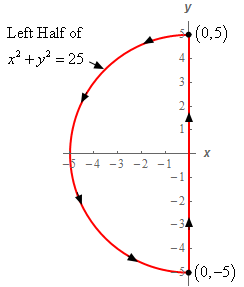Solution
2. Use Green’s Theorem to evaluate $$\displaystyle \int\limits_{C}{{\left( {6y - 9x} \right)dy - \left( {yx - {x^3}} \right)\,dx}}$$ where $$C$$ is shown below.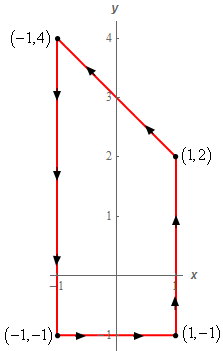Solution
3. Use Green’s Theorem to evaluate $$\displaystyle \int\limits_{C}{{{x^2}{y^2}\,dx + \left( {y{x^3} + {y^2}} \right)\,dy}}$$ where $$C$$ is shown below.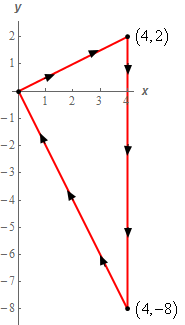Solution
4. Use Green’s Theorem to evaluate $$\displaystyle \int\limits_{C}{{\left( {{y^4} - 2y} \right)\,dx - \left( {6x - 4x{y^3}} \right)\,dy}}$$ where $$C$$ is shown below.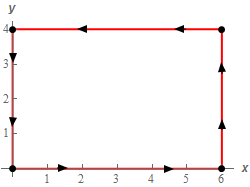Solution
5. Verify Green’s Theorem for $$\displaystyle \oint_{C}{{\left( {x{y^2} + {x^2}} \right)\,dx + \left( {4x - 1} \right)\,dy}}$$ where $$C$$ is shown below by (a)computing the line integral directly and (b) using Green’s Theorem to compute the line integral.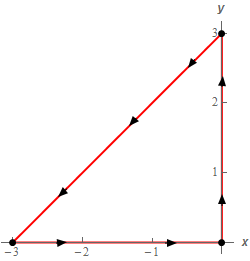Solution